EXPERIMENT NO.13

Dolph-Tchebyshev array

Practical Session Plan

 Time ( min) Content Learning Aid / Methodology Faculty Approach Typical Student Activity Skill / Competency Developed 10 Relevance and significance of experiment Chalk & Talk , Presentation Introduces, Facilitates, Monitors Listens, Participates, Discusses Knowledge, Communication, intrapersonal 20 Explanation of experiment Chalk & Talk , Presentation Introduces, Facilitates, Explains Listens Knowledge, Communication, intrapersonal, Application 20 Calculations N/A Explains, Monitors Participates, Discusses Knowledge, Communication, Intrapersonal, Application 60 Simulation Demonstration Explains, Monitors Participates, Discusses Debate, comprehension, Hands on experiment 10 Results and conclusions Keywords Lists, Facilitates Listens, Participates, Discusses Knowledge, Communication, intrapersonal, Comprehension

Title: Dolph-Tchebyshev array

Aim:

Â· To sketch the radiation pattern Dolph-Tchebyshev array.

Â· To compute performance parameters.

Objectives: Model Dolph-Tchebyshev array to analyze the performance parameters

Apparatus: PC, EM Simulation software

Theory:

coefficients are related to Tschebyscheff polynomials. A Dolph-Tschebyscheff array with no side lobes (or side lobes of âˆ’âˆž dB) reduces to the binomial design.

The recursion formula for Tschebyscheff polynomials is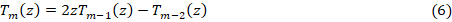It can be used to find one Tschebyscheff polynomial if the polynomials of the previous two orders are known. Each polynomial can also be computed using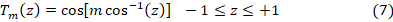The following properties of the polynomials are of interest:

1. All polynomials, of any order, pass through the point (1, 1).

2. Withinthe range âˆ’1 â‰¤ z â‰¤ 1, the polynomials have values within âˆ’1 to +1.

3. All roots occur within âˆ’1 â‰¤ z â‰¤ 1, and all maxima and minima have values of +1 an dâˆ’1, respectively.

Since the array factor of an even or odd number of elements is a summation of cosine terms whose form is the same as the Tschebyscheff polynomials, the unknown coefficients of the array factor can be determined by equating the series representing the cosine terms of the array factor to the appropriate Tschebyscheff polynomial. The order of the polynomial should be one less than the total number of elements of the array.

Procedure:

1. Click anywhere on the information window title bar and enter name of antenna you want to design and simulate then click Ok or press the <Enter> key.

2. Click anywhere on the selection button to its left. Enter desire frequency in the dialog text box, and click Ok or press <Enter>. It will automatically calculate wavelength and get displayed into wavelength menu.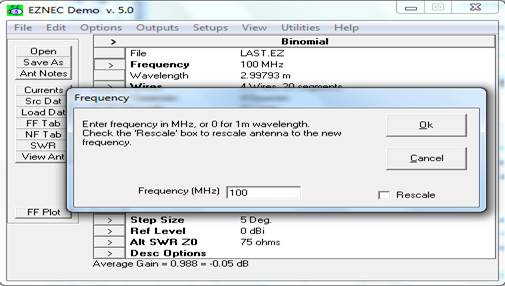3. Open the Wires Window by clicking on the Control Center "Wires" line, or the selection button to its left. Position the Wires and View Antenna Windows for convenient viewing. The position of each wire in space is specified by giving its x, y, and z coordinates. These coordinates represent distances from the origin.

e.g. for dipole that lies along X-axis at origin of length 0.5m has its X=0.25,Y=0,Z=0 (for end-1); X=-0.25,Y=0,Z=0 (for end-2). The last column is to define the number of segments for the wire. Total 20 segments are allowed for EZNEC-Demo version. As number of segments increases, accuracy of current distribution and thereby radiation pattern increases.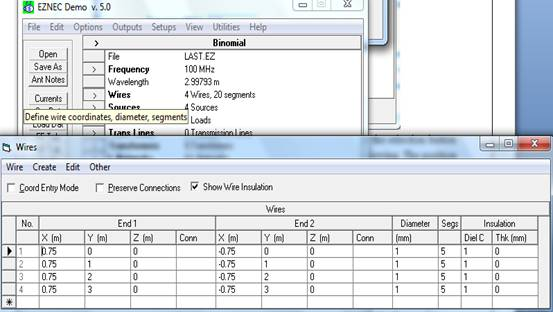4. In the Sources Window grid, you can see that there's one source (source no. 1), which is placed on wire #1, 50% from end 1. This is where we want the source to be. However, this is a good time to practice a little with the Sources Window. The source type, shown in the Type column, is a current source. The Sources Window also shows the source amplitude and phase, in this case one ampere at zero degrees. When a model contains only a single source, the magnitude, phase, or type have no effect on the pattern or source impedance.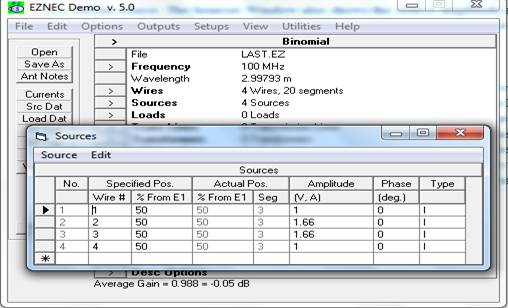5. Click on the Ground Type line, and select real. The height of the antenna will determine which ground model we use. If you have a fast computer, you can always choose High Accuracy analysis unless you need to make a connection to ground. When we changed the ground type to Real, one ground medium was created. The conductivity and dielectric constant of newly-created grounds can be set for your local ground conditions by means of the Control Center Options menu Default Ground Const selection. The values initially used by EZNEC are those of "average" or "good" ground (conductivity of 0.005 S/m, dielectric constant of 13).6. Click on the Units line or the selection button to its left, then select meter, and Ok.7. Click on the Plot Type row in the Control Center and select either of elevation, azimuth or 3D-pattern.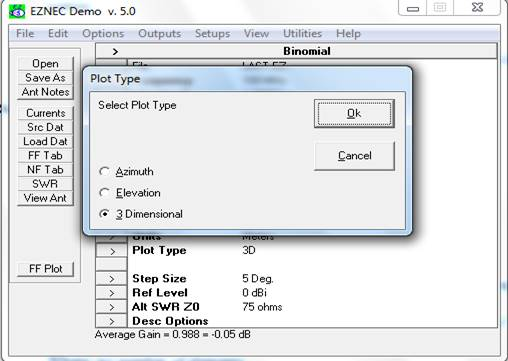8. Save the antenna description by clicking the Save As action button.

9.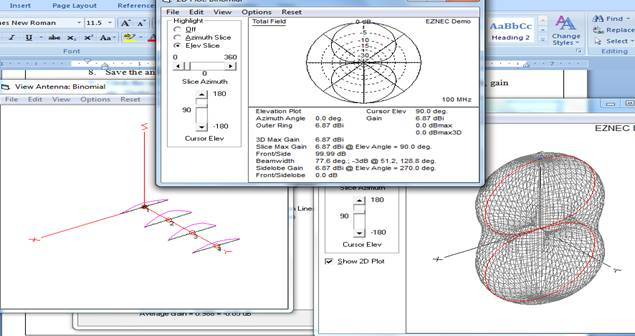Click the action button. FF Plot; it will show you the radiation pattern and beam width, gain etc. Under view option of 3D plot it is also possible to see 2D pattern of radiation.

Sample Calculations:

• Dimensions of array element (half wave dipole )

1. Calculate wavelength=>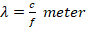2. Length of antenna=>meter.

3. Spacing between element=>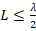• For Pascalâ€™s triangle

Calculate coefficient of binomial array (as amplitude of excitation) using following formulaWhere, n= number of elements.

Expected Output: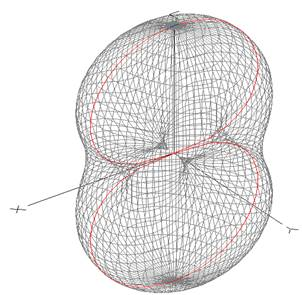Conclusion:

Dolph-Tchebysheff Array is based on Tchebysheff polynomials. It has narrowest main lobe for the given side lobe sides. The current distribution reduces the pattern which is similar to Tchebysheff polynomials.

Upon completion of experiment students will be able to :

Students will able to model the Dolph Tchebyshev, compute the performance parameters and compare the performance parameters with standard parameters

Oral Question Bank

Theory Question Bank

 Q. No Description 1. Define directivity? 2. Define antenna gain? 3. Define effective aperture? 4. What is collecting aperture? 5. Define HPBW? 6. Define FBR? 7. Define BWFN? 8. An array consisting a no. of equidistant dipoles of equal size, fed with the same currents and phase is called:(a) end fire array (b) back fire array (c) broad side array (d) binomial array 9. Choose the correct statement:(a) Both Binomial and uniform amplitude distributions are special cases of Dolph-Tchebyscheff distribution.(b) Both edge and uniform amplitude distributions are special cases of Dolph-Tchebyscheff distribution.(c) Both Binomial and edge istributions are special cases of the Dolph-Tchebyscheff (D-T) distribution.(d) All the three distributions listed in (a, b & c) above are special cases of Dolph-Tchebyscheff distribution 10. What is meant by Dolph Chebyshev array? 11. Define FSLBW? 12. What is the expression for FNBW for Dolph Chebyshev array? 13. What is the expression for HPBW for Dolph Chebyshev array? 14. Differentiate Dolph Chebyshev array and Binomial array? 15. What is the expression for minor lobe maxima for Dolph Chebyshev array? 16. What are the types of antenna arrays? 17. Define the length of an array? 18. What is array factor or space factor? 19. What are the applications of arrays? 20. What is a collinear array?